#### Integration-Midpoint and Simpsons Rules

Integration: Midpoint and Simpson’s Rules

Midpoint rule:

If we utilize the endpoints of the subintervals to approximate the integral we run the risk that the values at the endpoints don’t accurately represent the average value of the function on the subinterval. A point which is much more probable to be close to the average would be the midpoint of every subinterval.

Using the midpoint in the calculation is called the midpoint rule. On the i-th interval [xi−1, xi] we will call the midpoint x¯i that is
i=(xi-1 + xi)/2

If Δxi = xi− xi−1 is the length of each interval then using midpoints to approximate the integral would give the formula: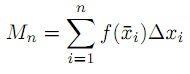For even spacing Δx = (b − a)/n as well as the formula is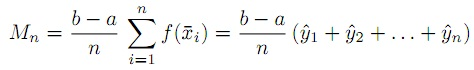where we define yˆi= f(x¯i).

While the midpoint method is clearly better than Lnor Rn it isn’t obvious that it is actually better than the trapezoid method Tn but it is.

Simpson’s rule:

If f isn’t linear on a subinterval then it can be seen that the errors for the midpoint and trapezoid rules behave in a very predictable way they have opposite sign. For illustration if the function is concave up then Tn will be too high, while Mn will be too low. Therefore it makes sense that a better approximation would be to average Tn and Mn. Nevertheless in this case we can do better than a simple average. The error will be minimized if we utilize a weighted average. To discover the proper weight we take advantage of the fact that for a quadratic function the errors EMn and ETnare exactly related by:
|EMn| =1/2|ETn|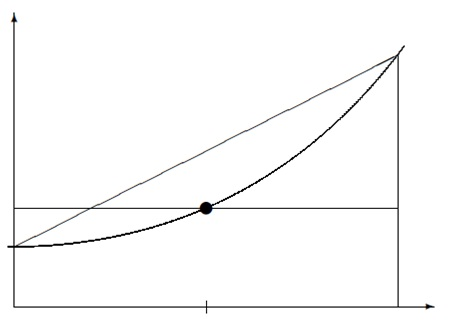Comparing the trapezoid as well midpoint method on a single subinterval. The function is concave up in that case Tn is too high while Mn is too low.

Consequently we take the following weighted average of the two which is called Simpson’s rule

S2n=2/3 Mn+1/3 Tn

If we utilize this weighting on a quadratic function the two errors will precisely cancel.

Notice that we inscribe the subscript as 2n. That is for the reason that we usually think of 2nsubintervals in the approximation the n subintervals of the trapezoid are additional subdivided by the midpoints. We are able to then number all the points using integers. If we number them this method we notice that the number of subintervals must be an even number.

The method for Simpson’s rule if the subintervals are consistently spaced is the following (with n intervals where n is even)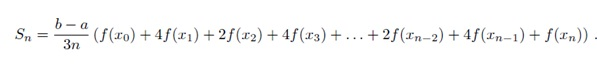Note that if we are presented with data {xi, yi} where the xipoints are evenly spaced with xi+1−xi= Δx,
it is simple to apply Simpson’s method: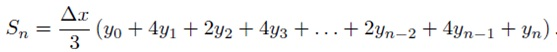Note the pattern of the coefficients. The following program will generate these coefficients for n intervals if n are an even number.

Try it for n = 67100.

function w = mysimpweights(n)
% calculate the weights for Simpson’s rule
% Input: n -- the number of intervals should be even
% Output: a vector with the weights, length n+1
if rem(n,2) ~= 0
error(’n must be even’)
end
w = ones(n+1,1);
for i = 2:n
if rem(i,2)==0
w(i)=4;
else
w(i)=2;
end
end

Simpson’s rule is incredibly precise. We will consider immediately how accurate in the next section. The one drawback is that the points utilized must either be evenly spaced or at least the odd number points must lie exactly at the midpoint between the even numbered points. In applications where you are able to choose the spacing this is not a problem. In applications such like experimental or simulated data you might not have control over the spacing and then you can’t use Simpson’s rule.

Error bounds:

The midpoint, trapezoid and Simpson’s rules are all approximations. As with any approximation prior to you can safely use it you should know how good (or bad) the approximation might be. For these methods there are formulas that provide upper bounds on the error. In other prose the worst case errors for the methods. These bounds are specified by the following statements:

• Suppose f′′ is continuous on [a,b]. Let K2 = maxx∈[a,b] |f′′(x)|. Afterwards the errors ETn and EMn of the Trapezoid and Midpoint rules respectively applied to ∫ba f dx satisfy: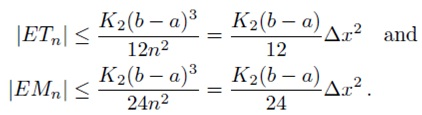• Presume f(4) is continuous on [a,b]. Let K4 = maxx∈[a,b] |f(4)(x)|. Afterwards the error ESn of Simpson’s rule applied to ∫baf dx satisfies: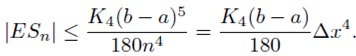In practice K2 also K4 are they approximated from the values of f at the evaluation points.

The most significant thing in these error bounds is the last term. For the trapezoid as well as midpoint method the error depends on Δx2 whereas the error for Simpson’s rule depends on Δx4. If Δx is now moderately small then there is a huge advantage with Simpson’s method.

While an error depends on a power of a parameter such as above we sometimes use the order notation. In the above error bounds we speak that the trapezoid and midpoint rules have errors of order O(Δx2) whereas Simpson’s rule has error of order O(Δx4).

In Mat lab there is a built-in command for definite integrals- quad (f, a, b) where the f is an inline function moreover a and b are the endpoints. Here quad rise for quadrature which is a term for numerical integration. The command utilizes ‘adaptive Simpson quadrature’ a form of Simpson’s rule that checks it’s possessed accuracy and adjusts the grid size where needed. Here is an instance of its usage

> f = inline(’x.^(1/3).*sin(x.^3)’)
> I = quad(f,0,1)

Latest technology based Matlab Programming Online Tutoring Assistance

Tutors, at the www.tutorsglobe.com, take pledge to provide full satisfaction and assurance in Matlab Programming help via online tutoring. Students are getting 100% satisfaction by online tutors across the globe. Here you can get homework help for Matlab Programming, project ideas and tutorials. We provide email based Matlab Programming help. You can join us to ask queries 24x7 with live, experienced and qualified online tutors specialized in Matlab Programming. Through Online Tutoring, you would be able to complete your homework or assignments at your home. Tutors at the TutorsGlobe are committed to provide the best quality online tutoring assistance for Matlab Programming Homework help and assignment help services. They use their experience, as they have solved thousands of the Matlab Programming assignments, which may help you to solve your complex issues of Matlab Programming. TutorsGlobe assure for the best quality compliance to your homework. Compromise with quality is not in our dictionary. If we feel that we are not able to provide the homework help as per the deadline or given instruction by the student, we refund the money of the student without any delay.